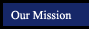Properties, Postulates and Theorems
textbook tutorials - definitions - constructions - postulates and theorems - internet activities and resources
 mathematical logic symmetry and similarity points, lines and planes parallel lines triangles quadrilaterals polygons circles 3-Dimensional Geometry
 Basic Geometry Concepts Coordinate Geometry Segment Addition Postulate The Distance Formula The distance between the points A(x1, y1) and B(x2,y2) is AB = square root of (x2 - x1)2 + (y2-y1)2. Unique Line Assumption The Midpoint Formula The midpoint of the segment joining the points A(x1, y1) and B(x2,y2)has the coordinates [(x1 + x2)/2 [(y1 + y2)/2]. The Slope Formula The slope m of the line containing the points (x1, y1) and (x2,y2) is m (y2 - y1) / (x2- x1). The equation of a line with slope m and y-intercept b is y = mx + b. Two nonvertical lines are parallel if and only if their slopes are equal. Two nonvertical lines are perpendicular if and only if the product of their slopes is -1. An equation of the circles with center (0,0) and radius r is x2 + y2 = r2. An equation of the circle with center (h,k) and radius r is (x - h)2 + (y - k)2 = r2.